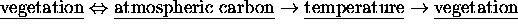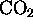Next: Introduction

# Climate, vegetation, and global carbon cycle: the simplest zero-dimensional model

Yuri M. Svirezhev and Werner von Bloh
Potsdam Institute for Climate Impact Research (PIK)
Telegrafenberg, P.O. Box 601203, D-14412 Potsdam
Germany

Ecological Modelling 101, 79-96 (1997)

### Abstract:

The mechanisms of interaction between climate and biosphere are studied for some hypothetical zero-dimensional (``point'' planet, where all parameters are globally averaged over the 2-dimensional surface of the planet, which is without ocean. These mechanisms are formed by two causal loops:and.

with a strong non-linear interaction. Using the conservation law for the total amount of carbon in the system and taking into account the assumption about quasi-stationary evolution of the system under anthropogenicemission, we reduce the dimension of the basic system of differential equations to 2. The reduced system is then studied by qualitative methods. The system can have up to five equilibria, three of them can be stable. Here there are two bifurcation parameters: total amount of carbon (A) and product of maximal plant productivity and residence time of carbon in the biota. Considering the system evolution under increase of A, we can observe the change of the planet ``status'' from ``cold desert'' to ``green cold planet'' (first bifurcation), then a "tropical planet'' arises (second bifurcation), and, as a result of further increase of carbon in the system, the planet transforms to a ``hot desert'' In conclusion the model was calculated for ``quasi-Earth'' values of parameters.

Keywords: Minimal model, biosphere, carbon cycle.Next: Introduction

Werner von Bloh (Data & Computation)
Thu Jul 13 11:24:58 MEST 2000